# COVID-19 SIR Modelling for Colombia

June 12, 2020

By  Edward Rodriguez

###### Move quickly from one page to another within COVID-19 Series from MATLAB Helper: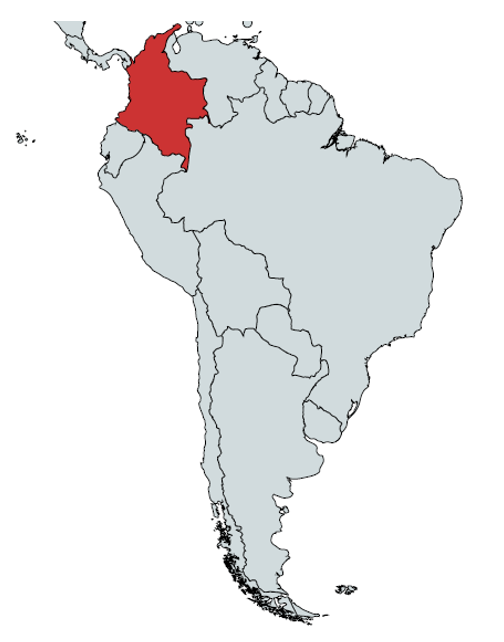We are starting with preprocessing data:

%url=http://datosabiertos.esri.co/datasets/colombia-covid19-coronavirus-casos-diarios/data

%% Read the excel file for covid cases (up to 22/05/20)

[row,col]= size(data);

time     = [1:1:row]';                     %days until today

n=100000;                                  %population

infected_real   = table2array(data(:,3));

recovered_real  = table2array(data(:,5));

susceptible_real=n-infected_real-recovered_real;

Using table2array, we can extract data for infected and recovered individuals.

## Estimating ???? and ????

The same approach for estimating ???? and ???? has been taken.

%% Computing beta and gamma

b=n*[0 diff(susceptible_real)']./(-1.*(susceptible_real.*infected_real));

b(b==inf) =[];

b(b==-inf)=[];

b(isnan(b)) = [];

b=reshape(b,[1,length(b)^2]);

b=median(b)

gamma=([0 diff(recovered_real)']./infected_real);

gamma(gamma==inf) =[];

gamma(gamma==-inf)=[];

gamma(isnan(gamma)) = [];

gamma=reshape(gamma,[1,length(gamma)^2]);

gamma=median(gamma)

Additionally, the reshape function was used for converting matrices values into vector values, and then, the median was computed.

b =

0.0766

gamma =

0.0085

## Building and Plotting SIR model

The SIR model is built in the model function

function dydt = model(t,y)

%y1 ->S(t)

%y2 ->I(t)

%y3 ->R(t)

n=100000;

beta=0.0766;

gamma=0.0085;

dydt = [-beta/n*y(1)*y(2);beta/n*y(1)*y(2)-gamma*y(2);gamma*y(2)];

end

Once the model is built, we plot the results. For this, we define initial conditions for the model with the CI variable. Then, Infected and recovered individuals are chosen to be shown.

%% Plotting model

CI=[susceptible_real(1);1;0];    %FOR S,I,R

time_span=[0 365];

[t,y] = ode45(@model,time_span,CI);

plot(t,y(:,1),t,y(:,2),t,y(:,3))

legend(["Susceptible";"Infected";"Recovered"])

title('SIR modeling')

xlabel('Days')

ylabel('Population')

## Results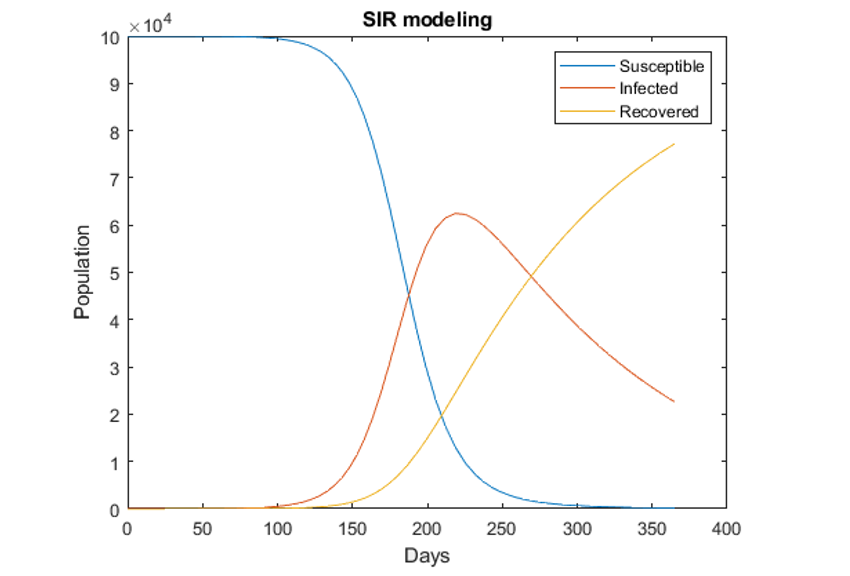Conclusion:

We have used data untill 31st May 2020 for all these analysis. We have seen some approaches to modelling diseases such as COVID-19 through MATLAB. We have found essential characteristics within this process. We have done Data Analysis and Data Extraction and included a reliable source for the COVID-19 data. We have used preprocessing to extract a subset of the collected data and organise the data accurately. We have done Data Modelling with model construction, i.e. finding the best model that fits the COVID-19 pandemic. We have done the calculation of model parameters using real collected data and finally done the Data Visualisation. All these steps were essential, and luckily, MATLAB has so many functions and tool to use in the given scenario.

Now summing our analysis and coming to a definite conclusion, India's COVID-19 case tally climbed from 100 to one lakh in just 64 days as per the data sourced from the Union Ministry of Health & Family Welfare (MoHFW). On comparing this with global data, sourced from Worldometers, it has been found out that India's COVID-19 growth rate is more than double the number of days as compared to Colombia.

### Important Note

Our COVID-19 codes and analysis have been made for educational and research purpose. We have shown different approaches of Pandemic Modelling for each state and the accuracy of result is not guaranteed for real-life situation. We wish for early end of this pandemic. Now it's in our hands; we need to take our responsibilities & take proper precautions.

Now let's have a quick view of the precautions.

#### Stay Home for non-essential activities!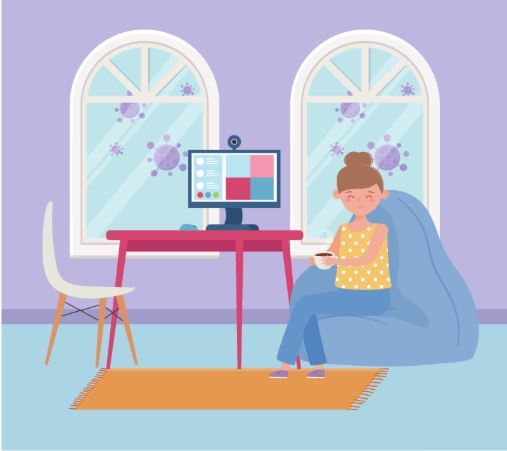Avoid going to crowded areas. Work From Home and Be A Super Hero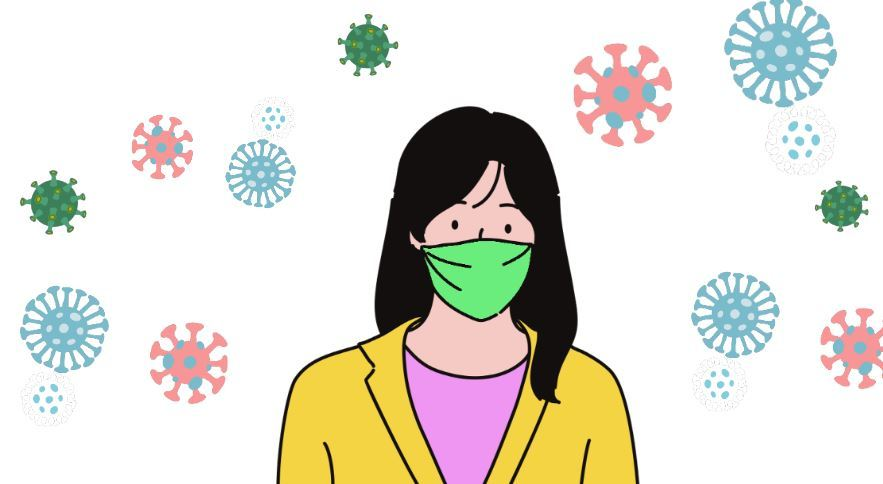Maintain distance while conversing with someone. Avoid touching your face frequently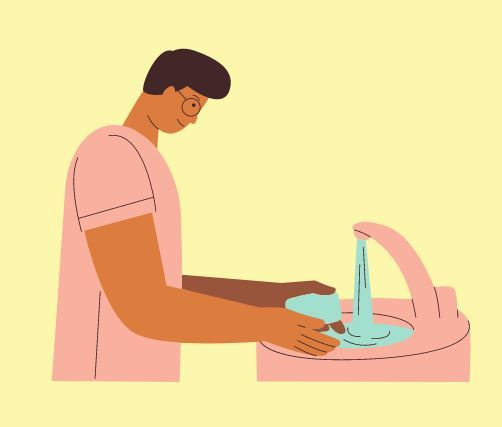Wash your hand for 20 seconds.  KEEP A SANTISER ALWAYS with you, wherever you go.While sneezing or coughing, always cover your mouth with a handkerchief or with your arm.

#### Home Quarantine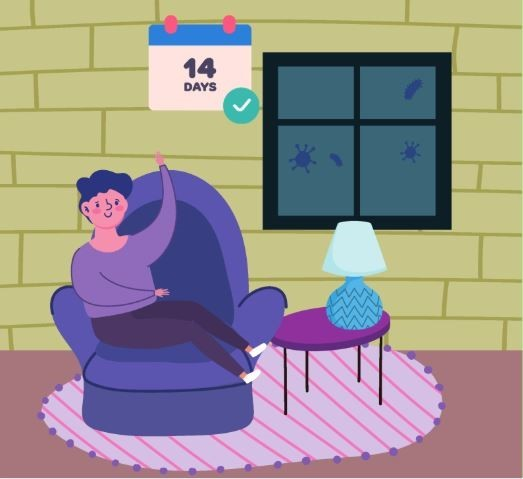If you have been facing the symptoms of Cold and Cough or the one's shown with COVID-19, then self-isolate yourself with home quarantine for 14 days.  So stay home stay safe; this is the best way you can contribute to the betterment of the nation.

#### Edward Rodriguez

My Name is Edward Rodriguez, I am 22 years old and I am from Cúcuta, Colombia (not Columbia). I studied Electronics Engineering at the Industrial of Santander University (UIS) in Bucaramanga, Colombia. I like listening to Salsa music and playing soccer in my free time!!!

•Nikita Mahoviya says:

Going through all the blogs of the COVID-19 analysis series by MATLAB Helper which were related to the various Indian states made me understand what the current situation is for India and now I came across the analysis for a different nation, Colombia, made me do a comparative study for both the countries.

Thank you, MATLAB Helper, for such valuable content at this time.

•If this code was written to address the case in Colombia, why the total population is supposed to be only 100,000? The point is, when the population is assumed to be a big number (such as the actual population size of the country), the answer goes beyond the rational range and seem s unreliable! Can you check the code with the real population?

•Edward Rodriguez says:

Hello Mahdi Shadab greetings! Well, about your doubt it is neccesary to consider that the whole population won’t be supossed susceptible! That means in any point of time the whole country population will not be infected

#### MATLAB Helper ®

Use Website Chat or WhatsApp at +91-8104622179